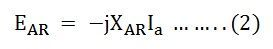# Synchronous Reactance and Synchronous Impedance

The Synchronous Reactance (XS) is the imaginary reactance employed to account for the voltage effects in the armature circuit produced by the actual armature leakage reactance and by the change in the air gap flux caused by the armature reaction.

Similarly, the Synchronous Impedance ZS is a fictitious impedance employed to account for the voltage effects in the armature circuit produced by the actual armature resistance, the actual armature leakage reactance, and the change in the air gap flux produced by the armature reaction.

The actual generated voltage consists of the summation of the two-component voltages. One of these component voltages would be generated if there were no armature reaction. It is the voltage that would be generated because of only the field excitation. This component of the generated voltage is called the Excitation Voltage (Eexc).

The other component of the generated voltage is known as the Armature Reaction Voltage (EAR). Thus, the two voltages that are the armature reaction voltage and the excitation voltage are added to keep a check on the effect of armature reaction upon the generated voltage. The equation is shown below.The voltage in a circuit caused by the change in the flux by the current is a result of armature reaction. The nature of this effect is inductive reactance. Therefore, EAR is equivalent to a voltage of inductive reactance and is given by the equation shown below.The Inductive Reactance XAR is a fictitious reactance. As a result a voltage is generated in the armature circuit. Therefore, armature reaction voltage can be modeled as an inductor in series with the internally generated voltage.

In addition to the effects of armature reaction, the stator winding also has a self-inductance and resistance.

Let,

• La is the self-inductance of the stator winding
• Xa is the self-inductive reactance of stator winding
• Ra is the armature stator resistance.

The terminal voltage V is given by the equation shown below.Where,

• RaIa is the armature resistance drop
• XaIa is the armature leakage reactance drop
• XARIa is the armature reaction voltage

The armature reaction and the leakage flux effects on the machine are both represented by inductive reactance. Therefore, all these combine to form a single reactance called Synchronous Reactance of the machine XS.Therefore,Where,The impedance ZS in the above equation (7) is the Synchronous Impedance, and XS is the Synchronous Reactance.